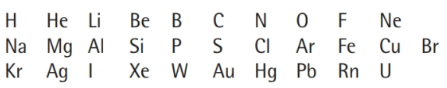# Use the periodic table to write the name and the atomic number of the element that corresponds to each of the following symbols:### Chemistry In Focus

7th Edition
Tro + 1 other
Publisher: Cengage Learning,
ISBN: 9781337399692

#### Solutions

Chapter
Section### Chemistry In Focus

7th Edition
Tro + 1 other
Publisher: Cengage Learning,
ISBN: 9781337399692
Chapter 3, Problem 5E
Textbook Problem
5 views

## Use the periodic table to write the name and the atomic number of the element that corresponds to each of the following symbols:Interpretation Introduction

Interpretation:

The name and the atomic number of the element that corresponds to each of the given symbols is to be written.

Concept Introduction:

The atomic number is the number of protons present in the nucleus of an atom.

An atom is the smallest unit of matter which cannot be further divided, and has all the characteristic properties of a chemical element.

An atom is the combination of outer electron cloud and a nucleus.

### Explanation of Solution

The name of the element represented by the symbol H, is hydrogen and the atomic number is 1.

The name of the element represented by the symbol He is helium and the atomic number is 2.

The name of the element represented by the symbol Li is Lithium and the atomic number is 3.

The name of the element represented by the symbol Be is beryllium and the atomic number is 4.

The name of the element represented by the symbol B is boron and the atomic number is 5.

The name of the element represented by the symbol C is carbon and the atomic number is 6.

The name of the element represented by the symbol N is nitrogen and the atomic number is 7.

The name of the element represented by the symbol O is oxygen and the atomic number is 8.

The name of the element represented by the symbol F is fluorine and the atomic number is 9.

The name of the element represented by the symbol Ne is neon and the atomic number is 10.

The name of the element represented by the symbol Na is sodium and the atomic number is 11.

The name of the element represented by the symbol Mg is magnesium and the atomic number is 12.

The name of the element represented by the symbol Al is aluminium and the atomic number is 13.

The name of the element represented by the symbol Si is silicon and the atomic number is 14.

The name of the element represented by the symbol P is phosphorous and the atomic number is 15.

The name of the element represented by the symbol S is sulfur and the atomic number is 16

### Still sussing out bartleby?

Check out a sample textbook solution.

See a sample solution

#### The Solution to Your Study Problems

Bartleby provides explanations to thousands of textbook problems written by our experts, many with advanced degrees!

Get Started

Find more solutions based on key concepts
Which of these foods should form the bulk of the pregame meal? a. breads, potatoes, pasta, and fruit juices. b....

Nutrition: Concepts and Controversies - Standalone book (MindTap Course List)

Step-by-step, explain how energy flows from the center of the Sun to Earth.

Horizons: Exploring the Universe (MindTap Course List)

What is physically exchanged during crossing over?

Human Heredity: Principles and Issues (MindTap Course List)

A tennis player receives a shot with the ball (0.060 0 kg) traveling horizontally at 20.0 m/s and returns the s...

Physics for Scientists and Engineers, Technology Update (No access codes included)